BATCH DISTILLATION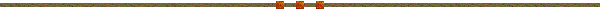This is a distillation step by step. This process don't start when the feed enter into the vessel (this is the main different with flash distillation), first the vessel is fill up to volume determinate. After that, it get heat, and the process start, until one of specific condition happen. This condition determinate the type of batch distillation

 Consideration and main equation The mixture R will be considerate in equilibrium with their vapors. The distillation start when the temperature in R, is bit more than its boil temperature, however this temperature is considerated equal boil temperature, and change because the composition in R change. The temperature in D is considerated equal to spray temperature in D. The velocity of this process depend of quantity of heat is giving to the vessels. The main equations is :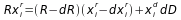Where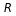are remain moles at time t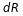is the variation of the remainder during the distillation and is equal dD from t to t+dt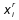the percentage molar of component i of the remainder at the time t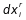are the masses of remainder that evaporate at the time t to t + dt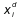is the percentage of component i in the distillate from time t to t + dt.

Despising insignificant terms and integrating, from initial time to t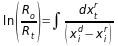where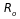are the masses of the remainder in the initial time.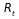are the masses of the remainder in the time t.is the percentage of component i of the remainder at the time tare the masses of remainder that evaporate in the time interval t to t + dtis the percentage of component i in the distillate at time t to t + dt.

In order to solve that equation you need to know this relation :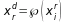is the percentage of the component i in the remainder at the time tis the percentage of the component i in the distillate at the time t to t + dt.

There are exists up to three type of batch distillation, classified according to this relation ()

1. Flash vaporization: (BATCH PROGRAM ONLINE)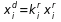is the percentage of the component i in the remainder at the time tis the percentage of the component i in the distillate at the time t to t + dt.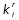is the equilibrium factor at time t

2. Batch Rectifier . It is classified in two subgroups
1. Reflux is constant and composition of the distillate is variable.
2. The composition of the distillate is constant and the Reflux is variable.

3. Batch Stripper o STRIPPING . used vapor, in order to purify organic compounds, it is classified in two :
1. Steam is condensed partially
2. Steam is not condensed.